### 3.4 Filters

Filters are a method for calculating a variety of useful quantities from the field variables as the solution evolves in time, such as time-averaged fields and extracting the field variables at certain points inside the domain. Each filter is defined in a `FILTER` tag inside a `FILTERS` block which lies in the main `NEKTAR` tag. In this section we give an overview of the modules currently available and how to set up these filters in the session file.

Here is an example `FILTER`:

1<FILTER TYPE="FilterName">
2    <PARAM NAME="Param1"> Value1 </PARAM>
3    <PARAM NAME="Param2"> Value2 </PARAM>
4</FILTER>

A filter has a name – in this case, `FilterName` – together with parameters which are set to user-defined values. Each filter expects different parameter inputs, although where functionality is similar, the same parameter names are shared between filter types for consistency. Numerical filter parameters may be expressions and so may include session parameters defined in the `PARAMETERS` section.

Some filters may perform a large number of operations, potentially taking up a significant percentage of the total simulation time. For this purpose, the parameter `IO_FiltersInfoSteps` is used to set the number of steps between successive total filter CPU time stats are printed. By default it is set to 10 times `IO_InfoSteps`. If the solver is run with the verbose `-v` flag, further information is printed, detailing the CPU time of each individual filter and percentage of time integration.

In the following we document the filters implemented. Note that some filters are solver-specific and will therefore only work for a given subset of the available solvers.

#### 3.4.1 Phase sampling

Note: This feature is currently only supported for filters derived from the FieldConvert filter: AverageFields, MovingAverage, ReynoldsStresses.

When analysing certain time-dependent problems, it might be of interest to activate a filter in a specific physical phase and with a certain period (for instance, to carry out phase averaging). The simulation time can be written as t = mT + nT T , where m is an integer representing the number of periods T elapsed, and 0 ≤ nT ≤ 1 is the phase. This feature is not a filter in itself and it is activated by adding the parameters below to the filter of interest:

 Option name Required Default Description `PhaseAverage` ✓ Feature activation `PhaseAveragePeriod` ✓ Period T `PhaseAveragePhase` ✓ Phase nT .

For instance, to activate phase averaging with a period of T = 10 at phase nT = 0.5:

1<FILTER TYPE="FilterName">
2    <PARAM NAME="Param1"> Value1 </PARAM>
3    <PARAM NAME="Param2"> Value2 </PARAM>
4    <PARAM NAME="PhaseAverage"> True </PARAM>
5    <PARAM NAME="PhaseAveragePeriod"> 10 </PARAM>
6    <PARAM NAME="PhaseAveragePhase"> 0.5 </PARAM>
7</FILTER>

Since this feature monitors nT every `SampleFrequency`, for best results it is recommended to set `SampleFrequency`= 1.
The maximum error in sampling phase is nT ,tol =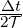`SampleFrequency`, which is displayed at the beginning of the simulation if the solver is run with the verbose `-v` option.
The number of periods elapsed is calculated based on simulation time. Caution is therefore recommended when modifying time information in the restart field, because if the new time does not correspond to the same phase, the feature will produce erroneous results.

#### 3.4.2 Aerodynamic forces

Note: This filter is only supported for the incompressible Navier-Stokes solver.

This filter evaluates the aerodynamic forces along a specific surface. The forces are projected along the Cartesian axes and the pressure and viscous contributions are computed in each direction.

The following parameters are supported:

 Option name Required Default Description `OutputFile` ✗ `session` Prefix of the output filename to which the forces are written. `Frequency` ✗ 1 Number of timesteps after which output is written. `Boundary` ✓ - Boundary surfaces on which the forces are to be evaluated.

An example is given below:

1  <FILTER TYPE="AeroForces">
2      <PARAM NAME="OutputFile">DragLift</PARAM>
3      <PARAM NAME="OutputFrequency">10</PARAM>
4      <PARAM NAME="Boundary"> B[1,2] </PARAM>
5  </FILTER>

During the execution a file named `DragLift.fce` will be created and the value of the aerodynamic forces on boundaries 1 and 2, defined in the `GEOMETRY` section, will be output every 10 time steps.

#### 3.4.3 Benchmark

Note: This filter is only supported for the Cardiac Electrophysiology Solver.

Filter `Benchmark` records spatially distributed event times for activation and repolarisation (recovert) during a simulation, for undertaking benchmark test problems.

1<FILTER TYPE="Benchmark">
2  <PARAM NAME="ThresholdValue"> -40.0 </PARAM>
3  <PARAM NAME="InitialValue">     0.0 </PARAM>
4  <PARAM NAME="OutputFile"> benchmark </PARAM>
5  <PARAM NAME="StartTime">        0.0 </PARAM>
6</FILTER>
• `ThresholdValue` specifies the value above which tissue is considered to be depolarised and below which is considered repolarised.
• `InitialValue` specifies the initial value of the activation or repolarisation time map.
• `OutputFile` specifies the base filename of activation and repolarisation maps output from the filter. This name is appended with the index of the event and the suffix ‘.fld‘.
• `StartTime` (optional) specifies the simulation time at which to start detecting events.

#### 3.4.4 Cell history points

Note: This filter is only supported for the Cardiac Electrophysiology Solver.

Filter `CellHistoryPoints` writes all cell model states over time at fixed points. Can be used along with the `HistoryPoints` filter to record all variables at specific points during a simulation.

1<FILTER TYPE="CellHistoryPoints">
2    <PARAM NAME="OutputFile">crn.his</PARAM>
3    <PARAM NAME="OutputFrequency">1</PARAM>
4    <PARAM NAME="Points">
5        0.00 0.0 0.0
6    </PARAM>
7</FILTER>
• `OutputFile` specifies the filename to write history data to.
• `OutputFrequency` specifies the number of steps between successive outputs.
• `Points` lists coordinates at which history data is to be recorded.

#### 3.4.5 Checkpoint cell model

Note: This filter is only supported for the Cardiac Electrophysiology Solver.

Filter `CheckpointCellModel` checkpoints the cell model. Can be used along with the `Checkpoint` filter to record complete simulation state and regular intervals.

1<FILTER TYPE="CheckpointCellModel">
2  <PARAM NAME="OutputFile"> session </PARAM>
3  <PARAM NAME="OutputFrequency"> 1 </PARAM>
4</FILTER>
• `OutputFile` (optional) specifies the base filename to use. If not specified, the session name is used. Checkpoint files are suffixed with the process ID and the extension ‘.chk‘.
• `OutputFrequency` specifies the number of timesteps between checkpoints.

#### 3.4.6 Checkpoint fields

The checkpoint filter writes a checkpoint file, containing the instantaneous state of the solution fields at at given timestep. This can subsequently be used for restarting the simulation or examining time-dependent behaviour. This produces a sequence of files, by default named `session_*.chk`, where `*` is replaced by a counter. The initial condition is written to `session_0.chk`. Existing files are not overwritten, but renamed to e.g. `session_0.bak0.chk`. In case this file already exists, too, the `chk`-file is renamed to `session_0.bak*.chk` and so on.

Note: This functionality is equivalent to setting the `IO_CheckSteps` parameter in the session file.

The following parameters are supported:

 Option name Required Default Description `OutputFile` ✗ `session` Prefix of the output filename to which the checkpoints are written. `OutputFrequency` ✓ - Number of timesteps after which output is written.

For example, to output the fields every 100 timesteps we can specify:

1<FILTER TYPE="Checkpoint">
2    <PARAM NAME="OutputFile">IntermediateFields</PARAM>
3    <PARAM NAME="OutputFrequency">100</PARAM>
4</FILTER>

#### 3.4.7 Electrogram

Note: This filter is only supported for the Cardiac Electrophysiology Solver.

Filter `Electrogram` computes virtual unipolar electrograms at a prescribed set of points.

1<FILTER TYPE="Electrogram">
2  <PARAM NAME="OutputFile"> session </PARAM>
3  <PARAM NAME="OutputFrequency"> 1 </PARAM>
4  <PARAM NAME="Points">
5      0.0  0.5  0.7
6      1.0  0.5  0.7
7      2.0  0.5  0.7
8  </PARAM>
9</FILTER>
• `OutputFile` (optional) specifies the base filename to use. If not specified, the session name is used. The extension ‘.ecg‘ is appended if not already specified.
• `OutputFrequency` specifies the number of resolution of the electrogram data.
• `Points` specifies a list of coordinates at which electrograms are desired. They must not lie within the domain.

#### 3.4.8 FieldConvert checkpoints

This filter applies a sequence of FieldConvert modules to the solution, writing an output file. An output is produced at the end of the simulation into `session_fc.fld`, or alternatively every M timesteps as defined by the user, into a sequence of files `session_*_fc.fld`, where `*` is replaced by a counter.

Module options are specified as a colon-separated list, following the same syntax as the `FieldConvert` command-line utility (see Section 5).

The following parameters are supported:

 Option name Required Default Description `OutputFile` ✗ `session.fld` Output filename. If no extension is provided, it is assumed as .fld `OutputFrequency` ✗ `NumSteps` Number of timesteps after which output is written, M. `Modules` ✗ FieldConvert modules to run, separated by a white space.

As an example, consider:

1<FILTER TYPE="FieldConvert">
2    <PARAM NAME="OutputFile">MyFile.vtu</PARAM>
3    <PARAM NAME="OutputFrequency">100</PARAM>
4    <PARAM NAME="Modules"> vorticity isocontour:fieldid=0:fieldvalue=0.1 </PARAM>
5</FILTER>

This will create a sequence of files named `MyFile_*_fc.vtu` containing isocontours. The result will be output every 100 time steps.

#### 3.4.9 History points

The history points filter can be used to evaluate the value of the fields in specific points of the domain as the solution evolves in time. By default this produces a file called `session.his`. For each timestep, and then each history point, a line is output containing the current solution time, followed by the value of each of the field variables. Commented lines are created at the top of the file containing the location of the history points and the order of the variables.

The following parameters are supported:

 Option name Required Default Description `OutputFile` ✗ `session` Prefix of the output filename to which the checkpoints are written. `OutputFrequency` ✗ 1 Number of timesteps after which output is written. `OutputPlane` ✗ 0 If the simulation is homogeneous, the plane on which to evaluate the history point. (No Fourier interpolation is currently implemented.) `Points ` ✓ - A list of the history points. These should always be given in three dimensions.

For example, to output the value of the solution fields at three points (1,0.5,0), (2,0.5,0) and (3,0.5,0) into a file `TimeValues.his` every 10 timesteps, we use the syntax:

1<FILTER TYPE="HistoryPoints">
2    <PARAM NAME="OutputFile">TimeValues</PARAM>
3    <PARAM NAME="OutputFrequency">10</PARAM>
4    <PARAM NAME="Points">
5        1 0.5 0
6        2 0.5 0
7        3 0.5 0
8    </PARAM>
9</FILTER>

#### 3.4.10 Kinetic energy and enstrophy

Note: This filter is only supported for the incompressible and compressible Navier-Stokes solvers in three dimensions.

The purpose of this filter is to calculate the kinetic energy and enstrophy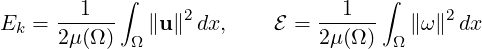where μ(Ω) is the volume of the domain Ω. This produces a file containing the time-evolution of the kinetic energy and enstrophy fields. By default this file is called `session.eny` where `session` is the session name.

The following parameters are supported:

 Option name Required Default Description `OutputFile` ✗ `session.eny` Output file name to which the energy and enstrophy are written. `OutputFrequency` ✓ - Number of timesteps at which output is written.

To enable the filter, add the following to the `FILTERS` tag:

1<FILTER TYPE="Energy">
2    <PARAM NAME="OutputFrequency"> 1 </PARAM>
3</FILTER>

#### 3.4.11 Modal energy

Note: This filter is only supported for the incompressible Navier-Stokes solver.

This filter calculates the time-evolution of the kinetic energy. In the case of a two- or three-dimensional simulation this is defined as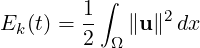However if the simulation is written as a one-dimensional homogeneous expansion so that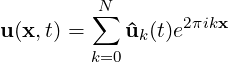then we instead calculate the energy spectrum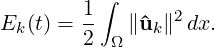Note that in this case, each component of ûk is a complex number and therefore N = `HomModesZ`∕2 lines are output for each timestep. This is a particularly useful tool in examining turbulent and transitional flows which use the homogeneous extension. In either case, the resulting output is written into a file called `session.mdl` by default.

The following parameters are supported:

 Option name Required Default Description `OutputFile` ✗ `session` Prefix of the output filename to which the energy spectrum is written. `OutputFrequency` ✗ 1 Number of timesteps after which output is written.

An example syntax is given below:

1<FILTER TYPE="ModalEnergy">
2    <PARAM NAME="OutputFile">EnergyFile</PARAM>
3    <PARAM NAME="OutputFrequency">10</PARAM>
4</FILTER>

#### 3.4.12 Moving body

Note: This filter is only supported for the Quasi-3D incompressible Navier-Stokes solver, in conjunction with the MovingBody forcing.

This filter `MovingBody` is encapsulated in the forcing module to evaluate the aerodynamic forces along the moving body surface. It is described in detail in section 11.3.4.1

#### 3.4.13 Moving average of fields

This filter computes the exponential moving average (in time) of fields for each variable defined in the session file. The moving average is defined as: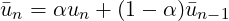with 0 < α < 1 and u1 = u1.

The same parameters of the time-average filter are supported, with the output file in the form `session_*_movAvg.fld`. In addition, either α or the time-constant τ must be defined. They are related by: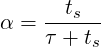where ts is the time interval between consecutive samples.

As an example, consider:

1<FILTER TYPE="MovingAverage">
2    <PARAM NAME="OutputFile">MyMovingAverage</PARAM>
3    <PARAM NAME="OutputFrequency">100</PARAM>
4    <PARAM NAME="SampleFrequency"> 10 </PARAM>
5    <PARAM NAME="tau"> 0.1 </PARAM>
6</FILTER>

This will create a file named `MyMovingAverage_movAvg.fld` with a moving average sampled every 10 time steps. The averaged field is however only output every 100 time steps.

#### 3.4.14 One-dimensional energy

This filter is designed to output the energy spectrum of one-dimensional elements. It transforms the solution field at each timestep into a orthogonal basis defined by the functions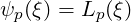where Lp is the p-th Legendre polynomial. This can be used to show the presence of, for example, oscillations in the underlying field due to numerical instability. The resulting output is written into a file called `session.eny` by default. The following parameters are supported:

 Option name Required Default Description `OutputFile` ✗ `session` Prefix of the output filename to which the energy spectrum is written. `OutputFrequency` ✗ 1 Number of timesteps after which output is written.

An example syntax is given below:

1<FILTER TYPE="Energy1D">
2    <PARAM NAME="OutputFile">EnergyFile</PARAM>
3    <PARAM NAME="OutputFrequency">10</PARAM>
4</FILTER>

#### 3.4.15 Reynolds stresses

Note: This filter is only supported for the incompressible Navier-Stokes solver.

This filter is an extended version of the time-average fields filter (see Section 3.4.16). It outputs not only the time-average of the fields, but also the Reynolds stresses. The same parameters supported in the time-average case can be used, for example:

1<FILTER TYPE="ReynoldsStresses">
2    <PARAM NAME="OutputFile">MyAverageField</PARAM>
3    <PARAM NAME="RestartFile">MyAverageRst.fld</PARAM>
4    <PARAM NAME="OutputFrequency">100</PARAM>
5    <PARAM NAME="SampleFrequency"> 10 </PARAM>
6</FILTER>

By default, this filter uses a simple average. Optionally, an exponential moving average can be used, in which case the output contains the moving averages and the Reynolds stresses calculated based on them. For example:

1<FILTER TYPE="ReynoldsStresses">
2    <PARAM NAME="OutputFile">MyAverageField</PARAM>
3    <PARAM NAME="MovingAverage">true</PARAM>
4    <PARAM NAME="OutputFrequency">100</PARAM>
5    <PARAM NAME="SampleFrequency"> 10 </PARAM>
6    <PARAM NAME="alpha"> 0.01 </PARAM>
7</FILTER>

#### 3.4.16 Time-averaged fields

This filter computes time-averaged fields for each variable defined in the session file. Time averages are computed by either taking a snapshot of the field every timestep, or alternatively at a user-defined number of timesteps N. An output is produced at the end of the simulation into `session_avg.fld`, or alternatively every M timesteps as defined by the user, into a sequence of files `session_*_avg.fld`, where `*` is replaced by a counter. This latter option can be useful to observe statistical convergence rates of the averaged variables.

This filter is derived from FieldConvert filter, and therefore support all parameters available in that case. The following additional parameter is supported:

 Option name Required Default Description `SampleFrequency` ✗ 1 Number of timesteps at which the average is calculated, N. `RestartFile` ✗ Restart file used as initial average. If no extension is provided, it is assumed as .fld

As an example, consider:

1<FILTER TYPE="AverageFields">
2    <PARAM NAME="OutputFile">MyAverageField</PARAM>
3    <PARAM NAME="RestartFile">MyRestartAvg.fld</PARAM>
4    <PARAM NAME="OutputFrequency">100</PARAM>
5    <PARAM NAME="SampleFrequency"> 10 </PARAM>
6</FILTER>

This will create a file named `MyAverageField.fld` averaging the instantaneous fields every 10 time steps. The averaged field is however only output every 100 time steps.

#### 3.4.17 ThresholdMax

The threshold value filter writes a field output containing a variable m, defined by the time at which the selected variable first exceeds a specified threshold value. The default name of the output file is the name of the session with the suffix `_max.fld`. Thresholding is applied based on the first variable listed in the session by default.

The following parameters are supported:

 Option name Required Default Description `OutputFile` ✗ session_max.fld Output filename to which the threshold times are written. `ThresholdVar` ✗ first variable name Specifies the variable on which the threshold will be applied. `ThresholdValue` ✓ - Specifies the threshold value. `InitialValue` ✓ - Specifies the initial time. `StartTime` ✗ 0.0 Specifies the time at which to start recording.

An example is given below:

1  <FILTER TYPE="ThresholdMax">
2      <PARAM NAME="OutputFile"> threshold_max.fld </PARAM>
3      <PARAM NAME="ThresholdVar"> u </PARAM>
4      <PARAM NAME="ThresholdValue"> 0.1 </PARAM>
5      <PARAM NAME="InitialValue">  0.4 </PARAM>
6  </FILTER>

which produces a field file `threshold_max.fld`.

#### 3.4.18 ThresholdMin value

Performs the same function as the `ThresholdMax` filter (see Section ??) but records the time at which the threshold variable drops below a prescribed value.